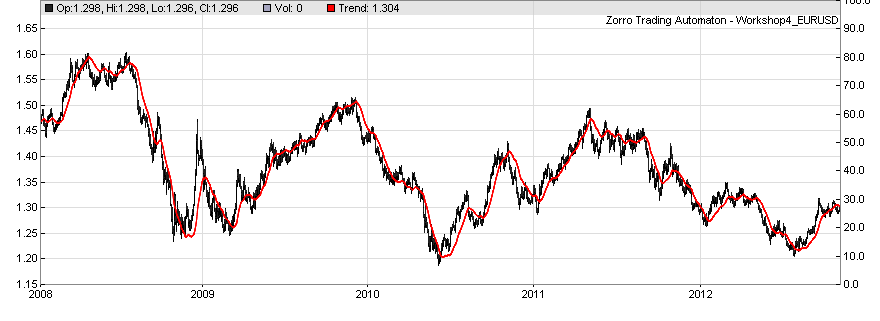# Workshop 4a: Indicator Implementation. More about series.

All the time, technical traders invent new indicators in their never ending hope to find one that really works. The article series Petra on Programming illustrates how to implement all sorts of complex and exotic indicators, and use them for trading systems. As an addendum to the previous workshop, we're going to implement the lowpass filter indicator. A second order lowpass filter is a bit more complex than its simple minded colleagues SMA, EMA, Stochastic, Ichimoku & Co. So it's a good exercise for serious indicator implementation.

When we're only interested in implementing an indicator from a ready formula, we can skip all math and start directly with the code. If you're interested in the theory, get the books by John Ehlers (book list) who introduced signal processing for traders. The Gaussian highpass filter formula below is from his book. For avoiding math, skip the following section.

### >>> Math starts here

A lowpass filter suppresses the high frequency components in a data stream, a highpass filter suppresses the low frequency components. The higher the order of the filter, the sharper is the frequency cutoff, which is normally desired for indicators. The filter's formula usually expresses the filter gain - output divided by input - in Z-transform notation. Here's the gain of a second order Gaussian highpass filter that you can get from a digital filter design book:

GainHP = Out / In = (1 - a/2)2 · (1 - 2 z-1 + z-2) / (1 - (2 - 2a) z-1 + (1 - a)2 z-2)

where a is the filter coefficient and the operator z-n applies n units of delay to the data. Z-transform notation is an elegant method to define arbitrary filters - if interested, read it up under the above link. For getting a lowpass filter, we can simply subtract the above highpass filter from a neutral filter with a gain of 1:

GainLP = Out / In = 1 - (1 - a/2)2 · (1 - 2 z-1 + z-2) / (1 - (2 - 2a) z-1 + (1 - a)2 z-2)

Converting to nominator / denominator form for separating input and output:

Out / In = (a - a2/4 + a2/2 z-1 - (a - 3a2/4) z-2) / (1 - (2 - 2a) z-1 + (1 - a)2 z-2)

Cross multiplication:

(1 - (2 - 2a) z-1 + (1 - a)2 z-2) · Out  = (a - a2/4 + a2/2 z-1 - (a - 3a2/4) z-2) · In

Applying the z operators to the input and output data; 1·Out = Out,  z-1·Out = Out, etc:

1 · Out - (2 - 2a) · Out + (1 - a)2 · Out = (a - a2/4) · In + a2/2 · In - (a - 3a2/4) · In

where Out is the current output value, Out is the output delayed by one bar, and so on. The final result:

Out = (a - a2/4) · In + a2/2 · In - (a - 3a2/4) · In + (2 - 2a) · Out - (1 - a)2 · Out

This is the formula that we can now directly implement as an indicator function.

### >>> Math ends here

With the series function that we already used in the previous workshop, it's easy to implement the delayed data from the above formula. The filter coefficient a is converted from a more convenient time period by a 'smoothing formula', normally a = 2/(1+Period). The higher the Period, the smaller is a and the stronger is the high frequency attenuation effect of the filter.

That's the implementation (Workshop4a):

```var lowpass(vars In,int Period)
{
var a = 2./(1+Period);
vars Out = series(In,3);
return Out = (a-0.25*a*a)*In
+ 0.5*a*a*In
- (a-0.75*a*a)*In
+ (2-2*a)*Out
- (1-a)*(1-a)*Out;
}
```

The first line converts the time period to the filter coefficient a. Mind the dot of the '2.'. The decimal point tells the compiler that it's a real number. A plain '2' would be an int. 1+Period is also an int, so we had an integer calculation that yields an integer result. Which will be normally 0 since the integer 2 divided by a higher integer is 0. This is one of the programming traps. If in doubt, put a decimal point to all numbers in any floating point formula in your scripts.

For the output we're creating a new series, but since we only need delay by of up to 2 bars, the series only needs a length of 3 elements. If we omitted the length parameter, the series had the length of the lookback period, which would be a waste of resources. We also initialize the series to the input data for preventing that it starts with 0.

Note that Period hs no effect on the amount of data. The filter uses only the last 3 bars. The plot of the lowpass function is the same as in the previous workshop, because the internal LowPass filter has the same formula:You can now look into the file indicators.c in the Source folder that contains the code of many indicators, and check out how those indicators work.

### What have we learned in this workshop?

• The Z-transform is a conventient way to define and calculate digital filters.
• Applying the Z transform to data is equivalent to taking a delayed element from a series.
• The length of a series can be given in the second argument.
• Mind the difference of integer and floating point calculations. Use decimal points when needed.
• Even complex filters need only a few lines of code.